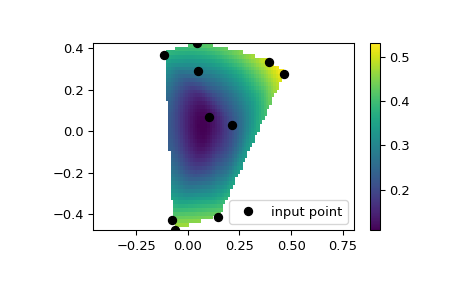# scipy.interpolate.CloughTocher2DInterpolator¶

class scipy.interpolate.CloughTocher2DInterpolator(points, values, tol=1e-06)

Piecewise cubic, C1 smooth, curvature-minimizing interpolant in 2D.

New in version 0.9.

Parameters
pointsndarray of floats, shape (npoints, ndims); or Delaunay

Data point coordinates, or a precomputed Delaunay triangulation.

valuesndarray of float or complex, shape (npoints, …)

Data values.

fill_valuefloat, optional

Value used to fill in for requested points outside of the convex hull of the input points. If not provided, then the default is nan.

tolfloat, optional

maxiterint, optional

Maximum number of iterations in gradient estimation.

rescalebool, optional

Rescale points to unit cube before performing interpolation. This is useful if some of the input dimensions have incommensurable units and differ by many orders of magnitude.

griddata

Interpolate unstructured D-D data.

LinearNDInterpolator

Piecewise linear interpolant in N dimensions.

NearestNDInterpolator

Nearest-neighbor interpolation in N dimensions.

Notes

The interpolant is constructed by triangulating the input data with Qhull , and constructing a piecewise cubic interpolating Bezier polynomial on each triangle, using a Clough-Tocher scheme [CT]. The interpolant is guaranteed to be continuously differentiable.

The gradients of the interpolant are chosen so that the curvature of the interpolating surface is approximatively minimized. The gradients necessary for this are estimated using the global algorithm described in [Nielson83] and [Renka84].

References

1

http://www.qhull.org/

CT

See, for example, P. Alfeld, ‘’A trivariate Clough-Tocher scheme for tetrahedral data’’. Computer Aided Geometric Design, 1, 169 (1984); G. Farin, ‘’Triangular Bernstein-Bezier patches’’. Computer Aided Geometric Design, 3, 83 (1986).

Nielson83

G. Nielson, ‘’A method for interpolating scattered data based upon a minimum norm network’’. Math. Comp., 40, 253 (1983).

Renka84

R. J. Renka and A. K. Cline. ‘’A Triangle-based C1 interpolation method.’’, Rocky Mountain J. Math., 14, 223 (1984).

Examples

We can interpolate values on a 2D plane:

>>> from scipy.interpolate import CloughTocher2DInterpolator
>>> import matplotlib.pyplot as plt
>>> np.random.seed(0)
>>> x = np.random.random(10) - 0.5
>>> y = np.random.random(10) - 0.5
>>> z = np.hypot(x, y)
>>> X = np.linspace(min(x), max(x))
>>> Y = np.linspace(min(y), max(y))
>>> X, Y = np.meshgrid(X, Y)  # 2D grid for interpolation
>>> interp = CloughTocher2DInterpolator(list(zip(x, y)), z)
>>> Z = interp(X, Y)
>>> plt.plot(x, y, "ok", label="input point")
>>> plt.legend()
>>> plt.colorbar()
>>> plt.axis("equal")
>>> plt.show()Methods

 Evaluate interpolator at given points.

#### Previous topic

scipy.interpolate.NearestNDInterpolator.__call__

#### Next topic

scipy.interpolate.CloughTocher2DInterpolator.__call__